Open in App
Not now

# Types Of Learning Rules in ANN

• Last Updated : 26 Oct, 2022

Learning rule enhances the Artificial Neural Network’s performance by applying this rule over the network. Thus learning rule updates the weights and bias levels of a network when certain conditions are met in the training process. it is a crucial part of the development of the Neural Network.

## Types Of Learning Rules in ANN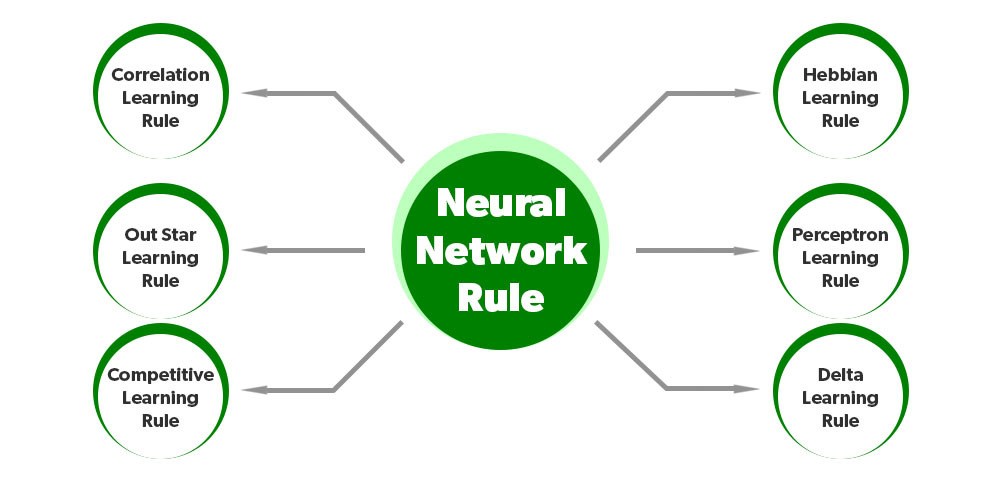### 1. Hebbian Learning Rule

Donald Hebb developed it in 1949 as an unsupervised learning algorithm in the neural network. We can use it to improve the weights of nodes of a network. The following phenomenon occurs when

• If two neighbor neurons are operating in the same phase at the same period of time, then the weight between these neurons should increase.
• For neurons operating in the opposite phase, the weight between them should decrease.
• If there is no signal correlation, the weight does not change, the sign of the weight between two nodes depends on the sign of the input between those nodes
• When inputs of both the nodes are either positive or negative, it results in a strong positive weight.
• If the input of one node is positive and negative for the other, a strong negative weight is present.

Mathematical Formulation:

`δw=αxiy`

where δw=change in weight,α is the learning rate.xi the input vector,y the output.

### 2. Perceptron Learning Rule

It was introduced by Rosenblatt. It is an error-correcting rule of a single-layer feedforward network. it is supervised in nature and calculates the error between the desired and actual output and if the output is present then only adjustments of weight are done.

Computed as follows:

Assume (x1,x2,x3……………………….xn) –>set of input vectors

and (w1,w2,w3…………………..wn) –>set of weights

y=actual output

wo=initial weight

wnew=new weight

δw=change in weight

α=learning rate

actual output(y)=wixi

learning signal(ej)=ti-y            (difference between desired and actual output)

δw=αxiej

wnew=wo+δw

Now, the output can be calculated on the basis of the input and the activation function applied over the net input and can be expressed as:

y=1, if net input>=θ

y=0, if net input<θ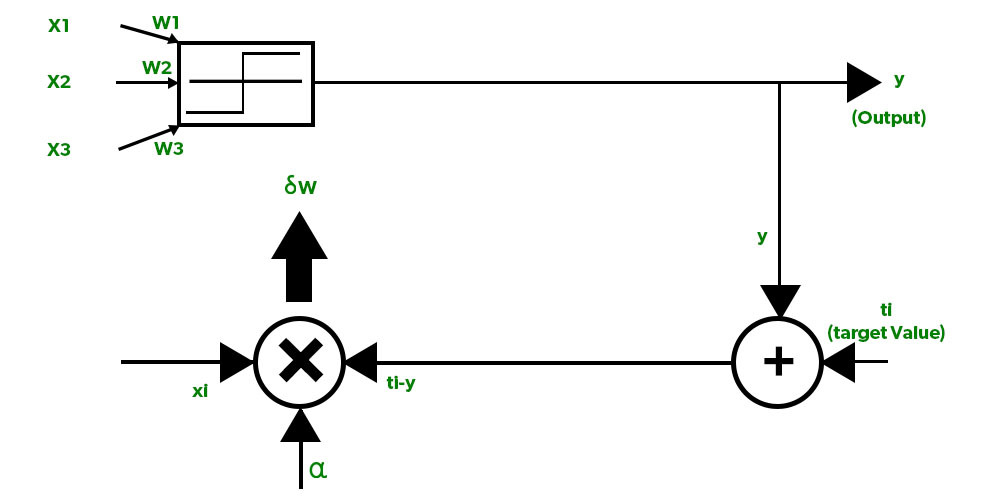### 3. Delta Learning Rule

It was developed by Bernard Widrow and Marcian Hoff and It depends on supervised learning and has a continuous activation function. It is also known as the Least Mean Square method and it minimizes error over all the training patterns.

It is based on a gradient descent approach which continues forever. It states that the modification in the weight of a node is equal to the product of the error and the input where the error is the difference between desired and actual output.

Computed as follows:

Assume (x1,x2,x3……………………….xn) –>set of input vectors

and (w1,w2,w3…………………..wn) –>set of weights

y=actual output

wo=initial weight

wnew=new weight

δw=change in weight

Error= ti-y

Learning signal(ej)=(ti-y)y’

y=f(net input)= ∫wixi

δw=αxiej=αxi(ti-y)y’

wnew=wo+δw

The updating of weights can only be done if there is a difference between the target and actual output(i.e., error) present:

case I: when t=y

then there is no change in weight

case II: else

wnew=wo+δw

### 4. Correlation Learning Rule

The correlation learning rule follows the same similar principle as the Hebbian learning rule,i.e., If two neighbor neurons are operating in the same phase at the same period of time, then the weight between these neurons should be more positive. For neurons operating in the opposite phase, the weight between them should be more negative but unlike the Hebbian rule, the correlation rule is supervised in nature here, the targeted response is used for the calculation of the change in weight.

In Mathematical form:

δw=αxitj

where δw=change in weight,α=learning rate,xi=set of the input vector, and tj=target value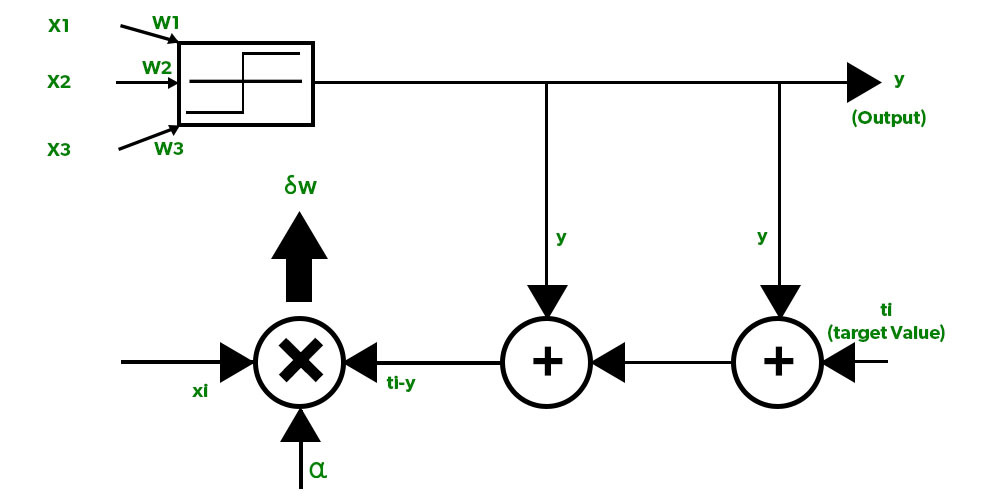### 5. Out Star Learning Rule

It was introduced by Grossberg and is a supervised training procedure.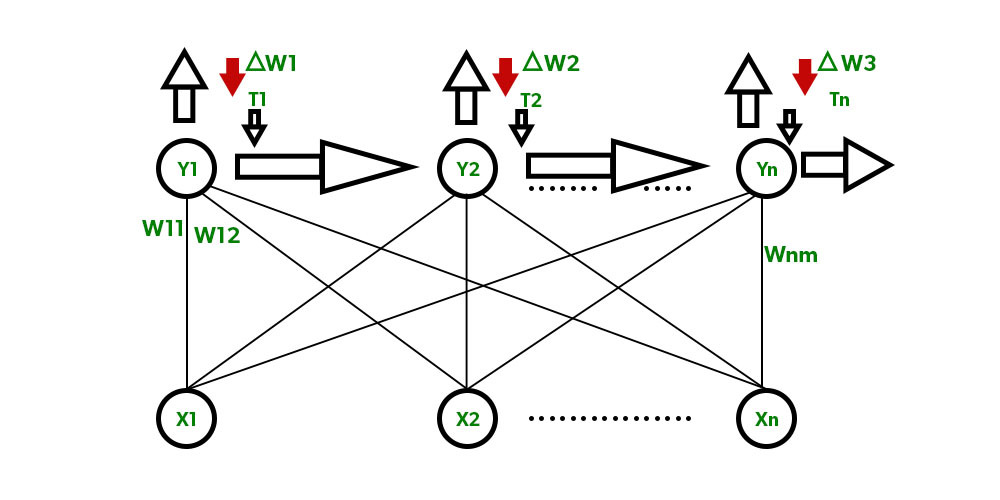Out Star Learning Rule is implemented when nodes in a network are arranged in a layer. Here the weights linked to a particular node should be equal to the targeted outputs for the nodes connected through those same weights. Weight change is thus calculated as=δw=α(t-y)

Where α=learning rate, y=actual output, and t=desired output for n layer nodes.

### 6. Competitive Learning Rule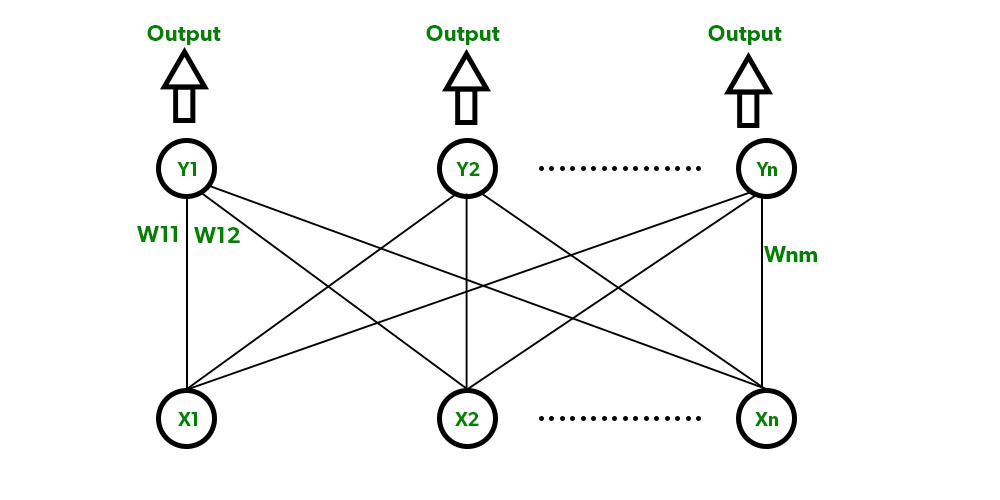It is also known as the Winner-takes-All rule and is unsupervised in nature. Here all the output nodes try to compete with each other to represent the input pattern and the winner is declared according to the node having the most outputs and is given the output 1 while the rest are given 0.

There are a set of neurons with arbitrarily distributed weights and the activation function is applied to a subset of neurons. Only one neuron is active at a time. Only the winner has updated weights, the rest remain unchanged.

My Personal Notes arrow_drop_up